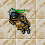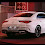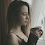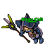## Wednesday, September 29, 2021

### TibiaGoals Riddles E12R06

Comment down below!

1.Tamarizz - Solidera

Move one arrow from the 8 to the 6, so the 8 would be a 9 and the 6 would be an 8
so we would have
9 + 9 = 18

2.3.4.9 + 9 = 18

Shizurie

5.6.9+9=18 Tris Merigold

7.There are no matchsticks, can't move any :P

/Yeka

8.Move the bottom left from the 8 to turn the 16 into 18

9+9=18
Xarkost Eilia

9.Bottom left vertical arrow of number 8 to the up left of number 6 so that the 8 forms a 9 and 6 forms an 8. 9+9=18

-Essiee

10.9 + 9 = 18

Righo

11.Move the arrow from the 8 (bottom left) to the 16 top right

9+9= 18

Skyelie Hasulhoff <3

12.You move the arrow from the bottom left of the eight over to the six and make the six and eight which makes nine plus nine equals eighteen.

Toxic Hasulhoff

13.14.9 + 9 = 18
Mian Stone'arrow

15.16.Psykeeh

9+9=18

17.18.You need to move arrow from 8 changing it to 9 and place it on 6 making 8 out og it.

9+9=18

/Yeka

19.9+9=18; Lord Neganek

20.We have to move 1 arrow from '8' lower/left side and put it on '16', then we have 9+9=18

Kenlux, Antica

21.Tynusiiaa/Antica

22.you can remove a arrow from the 8 making it 9 + 9 and placing the arrow on the 6 making it a 8 which would complete the equation 9 + 9 is 18

23.Turn the 8 into 9 & the 16 into 18
9 + 9 = 18

Char: Dyaniixz

24.character name: pablo reformed

25.character name: pablo reformed

26.27.9+9=18 /Norelli

28.9+9=18 Mikolaj

29.9+9= 18 .., frexiith

30.9+9= 18 .., frexiith

31.moving an arrow from number 8 (the lower left arrow) and placing it at number 16 (the upper right corner)
thus
9+8=16
will stay:
9+9=18
name: Archangel Rei

32.Heya! Move the lower left arrow of the 8 to the upper right of the 6 so the equation turns into 9+9=18.
Thanks a lot and have a nice day! :)

33.34.9+9=18
Lula Petit

35.Lula Petit
9+9=18

36.9 + 9 = 18
Character: Amy Meow

37.38.take an arrow off the 8 to make it 9, and put the arrow on the 6 to make it 8
resulting in 9+9=18
Griggi

39.9+9=18

Lovely olka

40.Congratulations to all participants!

You can control your results in our sheet: Riddles / Information

Remember, even if you got disqualified from the lottery, you can still participate in TibiaGoals riddles just for fun!

See you on Sunday!